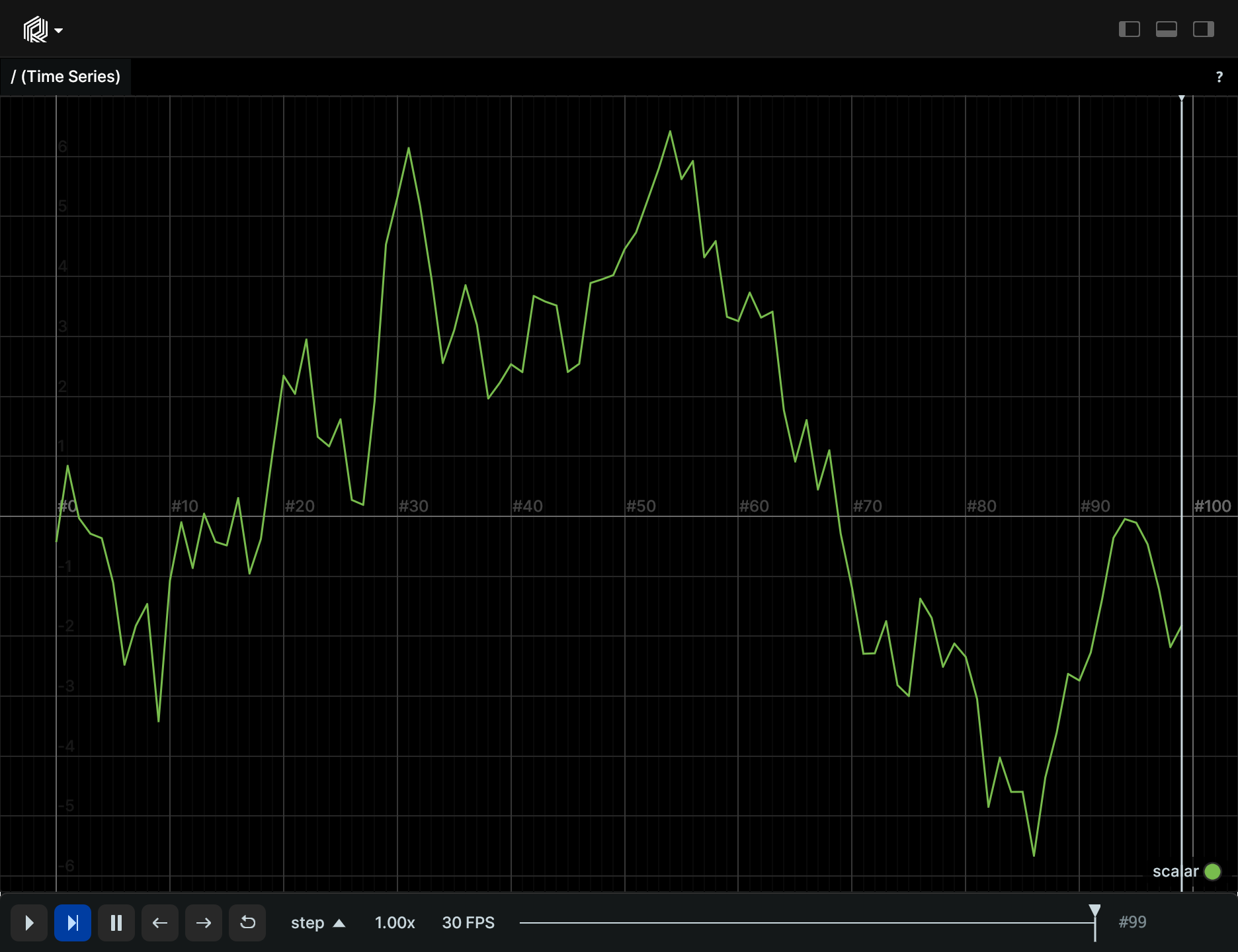# Scalar

`Scalar` represents a single scalar value that can be plotted as a time series if logged over time.

## Components and APIs

Primary component: `scalar`

Secondary components: `scalar_plot_props`, `colorrgba`, `radius`, `label`

Python APIs: log_scalar

Rust API: Scalar

## Simple Example

``````"""Log a scalar over time."""
import numpy as np
import rerun as rr

rr.init("rerun_example_scalar", spawn=True)
rng = np.random.default_rng(12345)

value = 1.0
for step in range(100):
rr.set_time_sequence("step", step)

value += rng.normal()
rr.log_scalar("scalar", value)
``````